# Current Electricity MCQ Level - 3

## 10 Questions MCQ Test Basic Physics for IIT JAM | Current Electricity MCQ Level - 3

Description
Attempt Current Electricity MCQ Level - 3 | 10 questions in 45 minutes | Mock test for Physics preparation | Free important questions MCQ to study Basic Physics for IIT JAM for Physics Exam | Download free PDF with solutions
QUESTION: 1

### The resistance of platinium wire at 0°C is 4Ω  What will be the resistance of the wire at 100°C. If the temperature coefficient of resistance of platinium is 0.0038/°C

Solution:

We know, Resistance depend on temperature by relation.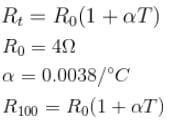R100 = 4(1 + 0.0038 × 100)
R100 = 4(1 + 0.38)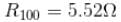QUESTION: 2

### Two metal ball of the same radius a  are located in homogeneous poorly conducting medium with resistivity ρ.The resistance of the medium between the ball provided that the separation between them is much greater than the radius of the ball.

Solution:

Let us impart charge  q  and  q  to the balls respectively. Potential difference between two balls

V = V+ – V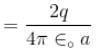Electric field at the surface of first sphere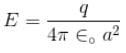electric field due to second sphere at the surface of first sphere is negligible
current density J = σE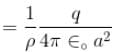The electric current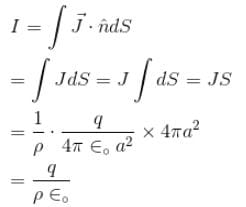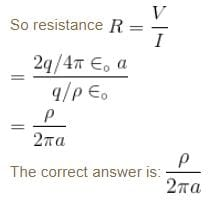QUESTION: 3

### In case of insulator as the temperature decrease resistivity

Solution:

QUESTION: 4

The law of refraction of direct current lines at the boundary between two conducting media having  σ1 and σ2  are the conductivity of media 1 and 2 and  α1 and α2 are the angle between the current lines and normal of the boundary surface as shown in figure.

Solution: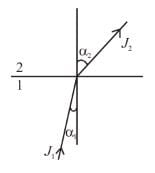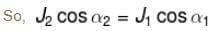Applying boundary condition of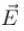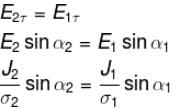Dividing (2) by (1)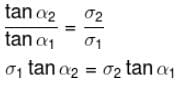The correct answer is: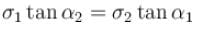QUESTION: 5

A metal ball of radius a  is surrounded by a thin concentric metal shell of radius b. The space between these electrodes is filled up with a poorly conducting homogeneous medium of resistivity ρ. The resistance of the interelectrode gap. assume  b → ∞

Solution:

As the current flow from inner to outer shell, the cross-section area increase. Assuming an element in form of spherical shell of radius r  and thickness  dr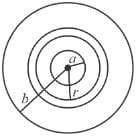The resistance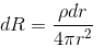Net resistance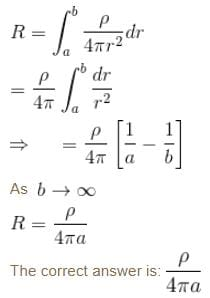QUESTION: 6

A long cylinder with uniformly charged surface an cross-section radius a  moves with a constant velocity v  along its axis. An electric field strength at the surface of the cylinder is equal to  E. So current caused by mechanical transfer of a charge.

Solution:

The convection current is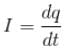Here, dq = λdx. where λ is the linear charge density. But, from the gauss, theorem, electric field at the surface of the cylinder,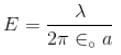Hence, substituting the value of λ and subsequently of dq  in Equation (i), we get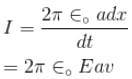The correct answer is: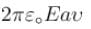QUESTION: 7

What is the drift velocity of an electron in a copper conductor having area 10 × 10–6 m2 and 10 × 1028 electron/m3

Solution:

We know, current through conductor = I = neAvd
where A = Area
vd = drift velocity
e = charge on electron
n = number of electron per unit volume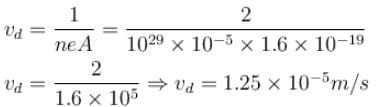The correct answer is: 1.25 × 10–5 m/s

QUESTION: 8

Two cylindrical conductor with equal cross-sections and different resistivity  ρ1 and ρ2 are put end to end. The charge at the boundary of the conductor if a current I flows from conductor 1 to conductor 2

Solution:

The current density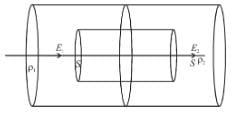J = I/A

Electric field in the two conductor are given by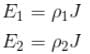If we consider of Gaussian pill box of cross-section area S at the interface of two conductors.

The flux of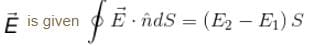According to Gauss Law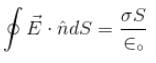where σ  is the surface charge density at the interface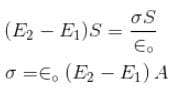So, charge at the boundary of two conductors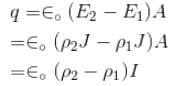The correct answer is: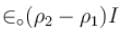QUESTION: 9

A current of 1A flows through a copper wire. How many electron pass through any cross-section of the wire in 1.6 seconds. (Charge on electron in 1.6 × 10–19C)

Solution:

I = 1A
t = 1.6 second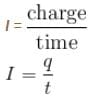⇒ q = It = 1 × 1.6 = 1.6
q = 1.6C
q = ne
n = number of electrons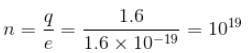QUESTION: 10

A battery of 6 cells, each of emf 2V and internal resistance 0.5Ω is being charged by a 220V  supplying  an external resistance of 10Ω is series. The potential difference across the battery is :

Solution:

Total emf of the battery = 12 V
Total internal resistance of the battery = 3Ω

Current through battery (I) =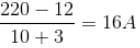Potential difference across battery  = emf of battery + Potential across the battery = 12 + (16 × 3)
= 60 V
The correct answer is: 60 VUse Code STAYHOME200 and get INR 200 additional OFF Use Coupon Code